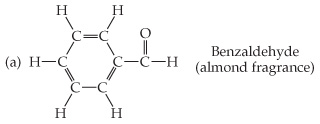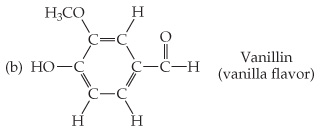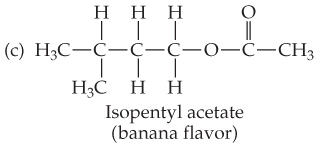# Problem: Based on the following structural formulas, calculate the percentage of carbon by mass present in each compound.

###### FREE Expert Solution

Benzaldehyde

= 106.118 g C7H6O

= 84.07 g C###### Problem Details

Based on the following structural formulas, calculate the percentage of carbon by mass present in each compound.What scientific concept do you need to know in order to solve this problem?

Our tutors have indicated that to solve this problem you will need to apply the Mass Percent Formula concept. You can view video lessons to learn Mass Percent Formula. Or if you need more Mass Percent Formula practice, you can also practice Mass Percent Formula practice problems.

What is the difficulty of this problem?

Our tutors rated the difficulty ofBased on the following structural formulas, calculate the pe...as low difficulty.

How long does this problem take to solve?

Our expert Chemistry tutor, Sabrina took 7 minutes and 54 seconds to solve this problem. You can follow their steps in the video explanation above.

What professor is this problem relevant for?

Based on our data, we think this problem is relevant for Professor Dixon's class at UCF.